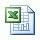# Starting volume of original mud (weight up with Barite)

You know how much volume will be increased due to adding barite into the system; however, you sometimes are limited to total volume due to limit pit volume on the rig so you need to calculate starting volume to achieve the predetermined final volume of desired mud weight. This formula below is used to determine the star volume of mud in case of weighting up with Barite.

Starting volume in bbl = VF x (35 – W2) ÷ (35 – W1)

Where; W1 = current mud weigth in ppg

W2 = new mud weight in ppg

VF = final volume in bbl

Example: Determine the starting volume (bbl) of 10.0 ppg (W1) mud required to achieve 100 bbl (VF) of 13.0 ppg (W2) mud with barite:

Starting volume in bbl = 100 x (35 – 13.0) ÷ (35 – 10.0)

Starting volume in bbl= 88.0 bbl

In order to achieve 100 bbl of 13.0 ppg mud weight up with barite, you must have 88.0 bbl starting volume of 10.0 ppg mud.

Please find the Excel sheetfor calculating Barrel of starting volume of original mud weight required to give a predetermined final volume of desired mud weight with barite.

### Related Post

Increase Mud Weight by Adding Hematite You have learn how to weight up with Barite andCalcium Carbonate from previous posts. Sometimes, you may need to weight up to extremely high weight th...
Boyle’s Gas Law and Its Application in Drilling Understand Boyle’s Gas Law Boyle’s law states that at constant temperature, the absolute pressure and the volume of a gas are inversely proportiona...
Drilling Formula Calculation Sheet Version 1 This is drilling formula calculation sheet v1 distributed on 28-Mar-10. I add many essential drilling formulas in to one Excel spread sheet and I woul...
Dilution of Mud System to Control Low Gravity Soli... By adding bbl of base fluid required, dilution of mud can help control Low Gravity Solid (LGS) in mud system. This post will demonstrate you how to de...
Share the joy
• 5
•
•
•
•
•
•
•
•
5
Shares